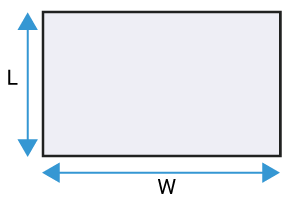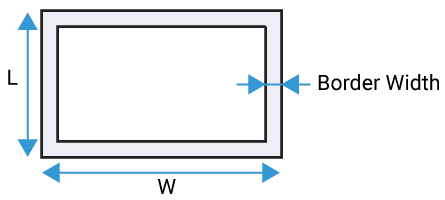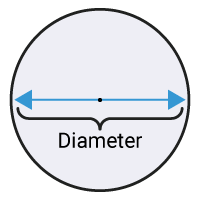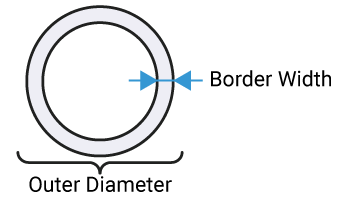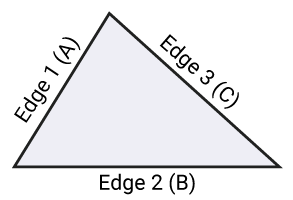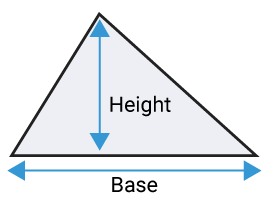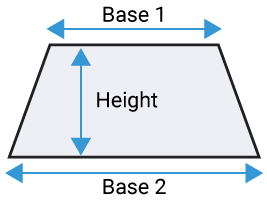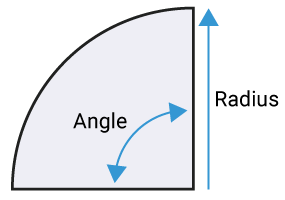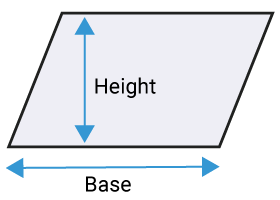## About Our Square Footage Calculator app

When we hear the term square footage calculator, the three questions that might rush into our mind would be below:

### What is the Square Footage?

Square footage is the measurement of a particular area that you end up with after calculating the square feet. Its abbreviation being sq.ft or ft(square).

### What is Square Footage Calculator?

A square footage calculator is a tool that calculates the result of square feet of space within a given area. Discover this square footage calculator app.

### How to Calculate Square Footage?

Multiplying the length and width of an area should give you the square footage.

Well, this and a lot more is what our square footage calculator tools do.

Why go for lengthy calculations when you have this square feet calculator.

### Perks of Using Our Square Footage Calculator.

In today’s world with state of the art technology, unlike the old days where all the buildings that we found were always in a perfect square shape, the possibilities of finding ample complex-shaped areas to work within our day to day lives aren’t seldom.

And sometimes the area that you wish to calculate might not be necessary in a square or rectangle shape, it is possible to have an oddly shaped area to work with.

This is when our sq ft calculator comes handy because what square footage calculator does is to assist you with calculating the square footage of any area in an instant, thus giving you the exact result that you need to know about the square footage.

### Benefits of Square Footage Calculator.

The benefit of a square footage calculator inches is that they make all the hard work easy on you. Yes, the very use of square footage calculation by assisting you with our exemplary square footage calculator app.

Square footage calculator needs to be used in our day to day lives to calculate a space that we use or need to use like construction, tiling, painting, gardening, hard flooring, drywalling and so on.

### How to Use Our Square Footage Calculator Tool?

Let’s take a look at the types of areas that we might need to calculate on a day to day basis.

• Rectangle
• Circle
• Triangle

These are the common types of areas for which we might require to calculate the square footage area.

To begin with, what you would need to do first is convert the measurement into square feet.

Rectangle

sq. ft. = l × w

l = length

w = width

Circle

sq. ft. = πr2

π = 3.14159265359

Triangle

s = 1/2(a + b + c)

sq. ft. = s(s – a)(s – b)(s – c))

a = edge a

b = edge b

c = edge c

For quick answers, use this triangle square footage calculator.

And then use our square foot calculator to calculate the square footage.

Below is a conversion table given to convert square inches, square centimetres, square feet, square yards, square meters and square millimetres along with the abbreviations of unit area for your convenience.

 Square feet to yards multiply ft2 by 0.11111 to get yd2 Square feet to meters multiply ft2 by 0.092903 to get m2 Square yards to square feet multiply yd2 by 9 to get ft2 Square yards to square meters multiply yd2 by 0.836127 to get m2 Square meters to square feet multiply m2 by 10.7639 to get ft2 Square meters to square yards multiply m2 by 1.19599 to get yd2 Square meters to square millimetres multiply the m2 value by 1000000 to get mm2 Square meters to square centimetres multiply the m2 value by 10 000 to get cm2 Square centimetres to square metres multiply the cm2 value by 0.0001 to get mm2 Square centimetres to square millimetres multiply the cm2 value by 100 to get mm2 Square millimetres to square centimetres multiply the mm2 value by 0.000001 to get cm2 Square millimetres to square metres multiply the mm2 value by 1000000 to get m2

#### Abbreviations of unit area

Ft2 = Square feet

In2 = Square Inches

Yd2 = Square Yards

Cm2 = Square Centimeters

Mm2 = Square Millimeters

M2 = Square Meters

History